# 1350 Limits

1350 Limits

## Math 1350 - Limits and continuity

MAPLE has a powerful capability to find a limit. The MAPLE function that is used to find a limit is limit.

Example1:

find the limit of f(x) = (x-2)/(x^2-1)

First define the function f(x)

> f:=x->(x-2)/(x^2 - 1);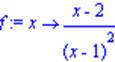Find the limit of f(x) as x approaches 0, 1, -1, infinity

> limit(f(x), x=0);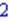> limit(f(x), x=1);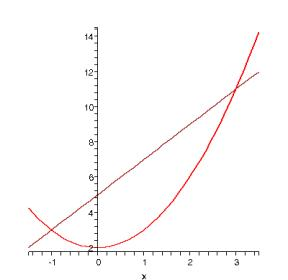> limit(f(x), x=-1);> limit(f(x), x=infinity);When x approaches 1, -1 the limit does not exist therefore MAPLE gives undefined.

To convince ourselves about the function´s behavior we can plot it.

> plot(f(x), x=-10..10, y=-10..10);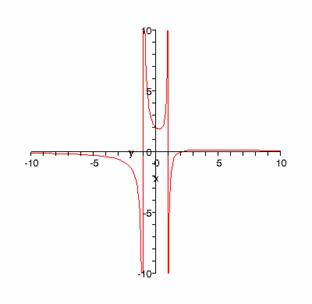Example2:

a different behavior has the limit of f(x) = (x-2)/(x-1)^2.

> f:=x->(x-2)/(x - 1)^2;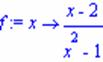> limit(f(x), x=0);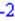> limit(f(x), x=1);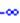> limit(f(x), x=-1);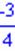> limit(f(x), x=infinity);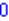> plot(f(x), x=-10..10, y=-10..10);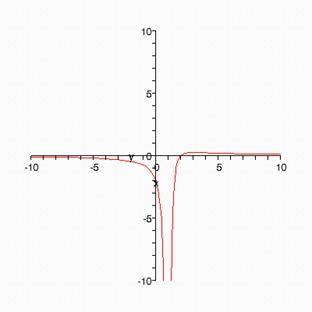Example3:

find the limit of f(x)=|x| as x -> 0. To write in MAPLE the absolute value of x, use the command abs.

> f:=x->abs(x);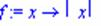> limit(f(x), x=0);Example3:

define f(x) = sin(1/x). Find the limit as x -> 0, and as x -> infinity. Plot the function.

> f:=x->sin(1/x);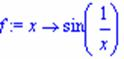> limit(f(x), x=0);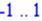> limit(f(x), x=infinity);> plot(f(x), x=-10..10, y=-1.5..1.5);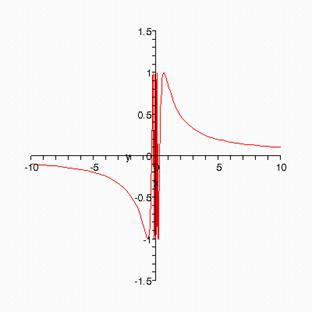f(x) is not continuous at as x -> 0 because its limit is ?1..1. It is continuous everywhere else since limit f(x) = 0 as x -> .

Exercises:

Find the limit of the fallowing functions a) as x -> 0; b) as x->. Plot them.

1. f(x) = (e^x 1)/x
2. f(x) = (e^x 1)/e^x
3. f(x) = 5-2*x
4. f(x) = ln(x)
5. f(x) = cos(x)
6. f(x) = (1/x)*cos(x)
7. f(x) = (1/x)*sin(1/x)